# Traveling Waves

If we recall the most general expression for the phase of an oscillator, we see at once that waves can also travel:

y(x,t) = A sin (kx + ωt + δ).
To simplify things, we will set the phase angle equal to zero and rewrite this equation as
y(x,t) = A sin (k (x + (ω/k)t)).
Compare this to the form of a function which undergoes translation: the transformation
y(x) -> y(x + a)
moves the function to the left a units: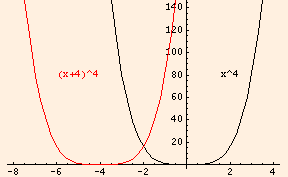This shows us that our traveling wave is simply an ordinary sine wave which is being translated by an amount depending on time. As we saw in the last section, w/k is the speed of the wave which in this case is traveling to the left: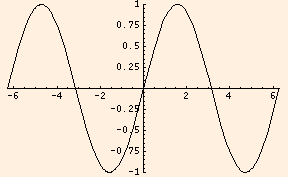A wave which travels to the right is described by

y(x,t) = A sin (kx - ωt).
We say that a traveling wave propagates through the medium. Sound is a longitudinal wave: the wave function describes changes in air pressure which are parallel to the direction the wave is traveling. Water waves, seismic waves and light waves are all transverse: the displacement of the water, ground or electric and magnetic fields are all perpendicular to the direction the wave is traveling. The foremost edge of a traveling wave is called the wave front, and plays an important role in our understanding of wave behavior.

### Huygens' Principle

Huygens' Principle states that each point on a wave front acts like a source for the next wave front. It is as if there is a tiny harmonic oscillator at every point in space and the vibration of one oscillator along a traveling wave causes the next one in the direction of travel to begin vibrating: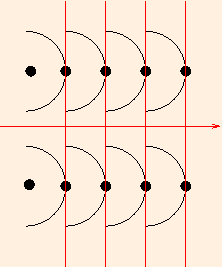Each oscillator generates a hemispherical wave disturbance in the direction of travel, which we have shown here in cross section as semicircles. The wave front is tangent to the semicircles at any given time.

We found that the energy of an oscillator is proportional to the square of its amplitude. For a spherical wave, that energy is evenly distributed on the surface of an ever-expanding sphere. We define the intensity to be the ratio of the power carried by the wave to the surface area of the spherical wave front:
I = P / (4 π r2).
From this we can see that while the total energy in the wave is constant (ignoring dissipative effects), the intensity drops off as 1 / r2.
Consider the reflection of an image in a mirror. For simplicity, we will follow just two rays of light from the image, showing the incoming wave fronts in red and the outgoing wave fronts in blue at equal time intervals: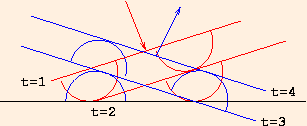We see that the angle of reflection is equal to the angle of the incident rays.

v = c / n,
n is called the index of refraction; it is always greater than 1 and so the speed of light in any material medium is always less than the speed of light in a vacuum. In general, the index of refraction is a function of wavelength; for sound,
• n = 1 in air;
• n = .06 in glass;
• n = .23 in water;
• n= 6.1 in rubber;
while for light (of wavelength 589.3 nm),
• n = 1 in a vacuum;
• n = 1.0003 in air;
• n = 1.333 in water;
• n = 1.336 in vitreous humour (inside the eye);
• n = 1.413 in the eye's lens;
• n = 1.52 in crown glass;
• n = 1.61 in flint glass, and
• n = 2.42 in diamond.
Since the speed of light is less in water than in air, the wave fronts change direction when crossing from one medium to the other: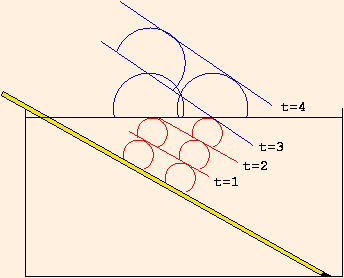n1 sin θ1 = n2 sin θ2
where the angles are measured from the normal to the interface between the two media: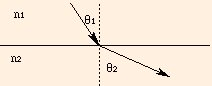θ c = arcsin (n 2 / n 1)
Snell's Law is not applicable; instead of refraction, there is total internal reflection, which means that the ray (and hence the wave) does not cross the boundary.

It is the refraction of light which makes possible both vision and vision correction. A ray of light as it traverses corrective lenses and the eye is refracted:

1. as it enters the corrective lens;
2. as it exits the corrective lens;
3. as it enters the cornea;
4. as it exits the cornea into the eye's lens;
5. and finally the ray is refracted as it exits the eye's lens and enters the vitreous humour.
The depth, shape and index of refraction of each medium determines the degree to which the rays of light are bent, and hence the focal point: where the image is in focus. The focal point should be just short of the retinal surface; if it is too close to the eye's lens, the eye is near-sighted and requires a divergent, defocusing corrective lens; if it is on the retinal surface, the world appears as a bright point of light; and if it is behind the retinal surface, the eye is far-sighted and requires a convergent, focusing corrective lens. Careful application of Snell's Law will enable you to determine whether concave or convex lenses are focusing or defocusing.

### Interference

Recall the wave demonstration applet in the previous section. Set the black and blue waves to the same mode number and observe the red superposition wave as you vary the blue wave phase angle. When the phase angle is zero, the black and blue waves are said to be in phase and constructively interfere. When the phase angle is π or -π, the superposition wave has an amplitude of zero and the black and blue waves are said to be out of phase: they destructively interfere. The interference of coherent waves (which start out in phase) lies at the heart of the quintessential wave phenomenon: diffraction.

We start by illustrating how interference works in two dimensions with this applet, which shows the superposition of two waves from sources at the bottom of the image. The white spots are where the waves constructively superpose and are in phase, and the black spots are where the waves destructively superpose and are out of phase:

You need a Java-capable browser to be able to use the applets. If they do not work with your Windows system, download the Java VM (Virtual Machine) for your version of Windows at the download section at java.sun.com.

Suppose that a beam of light is incident on a surface with two slits in it (separated by a distance d). Huygens' principle states that the two slits will act as coherent sources. These correspond to the applet above, with equal frequencies and zero phase angle. When the light rays from these two sources interfere at the screen (a distance L away from the slits), one will have traveled farther than the other: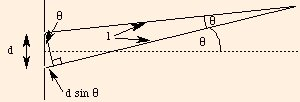The angles are approximately equal, as are the hypotenuse and adjacent sides of the large triangle, if the angle is small. This is true since the length L is usually much greater than the distance between the slits. The difference in length of the two rays from the slits to their point of intersection is d sin θ. When this length difference is an integral number of wavelengths:

d sin θ = m λ,
the phase difference is
k Δx = (2 π / λ) * m λ
= 2 π m,
so the waves are in phase, and we have constructive interference and a bright spot at the point of intersection (at x = L sin θ). When this length difference is a wavelength times an integer plus one half:
d sin θ = (m + 1/2) λ,
the phase difference is
k Δx = (2 π / λ) * (m + 1/2) λ
= 2 π m + π,
so the waves are π radians out of phase, and we have destructive interference with a dark spot at the intersection. If we label the minima and maxima with a number "a" as follows: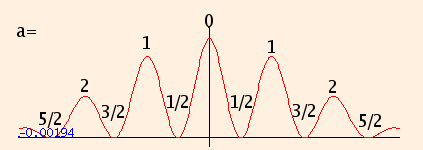we can write their locations as

x = a L λ / d.
The first applet plots the intensity as a function of position, while the second applet provides a visual illustration of the actual diffraction pattern:

You need a Java-capable browser to be able to use the applets. If they do not work with your Windows system, download the Java VM (Virtual Machine) for your version of Windows at the download section at java.sun.com.

While we have used light waves in this section to describe reflection, refraction and diffraction, is important to realize that sound waves share in all of these behaviors. For us, the primary difference between the two is simply their speeds of propagation. We can compute the positions of maxima and minima of light intensity given the geometry of the experiment, the frequency of the wave and the index of refraction of the medium:

You need a Java-capable browser to be able to use the applets. If they do not work with your Windows system, download the Java VM (Virtual Machine) for your version of Windows at the download section at java.sun.com.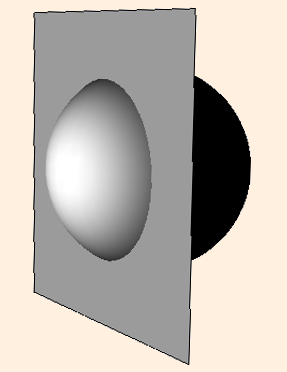For a convex mirror (looking into the outside of the spherical segment), the magnification of the image (the ratio of the image size to the object size) is equal to 1 when the object touches the mirror, and reduces to 0 as the object recedes to infinity; and the image is always "right-side-up".

But for a concave mirror (looking into the inside of the spherical segment), something very interesting happens. Huygens' Principle leads us to discover that the magnification M is given by

M = 1 / (1 - 2 O / r)
where O is the distance from the object to the surface of the mirror. M is still 1 when the object touches the mirror, and the image is still right-side-up. But as the object recedes toward a distance r/2 from the mirror, the magnification approaches infinity (here, r = 2m):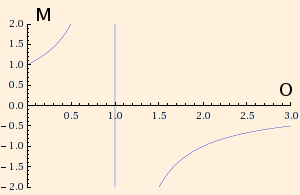As the object continues to recede, the image is inverted (M < 0), and slowly shrinks in magnification. Try this with the inside of a highly polished serving spoon, or some other reflecting concave surface.

ds2 = dr2 / (1 - 2 M / r)
Here, as an object approaches r=2M, a far-away observer sees any change in position as taking infinitely long: the inward-falling object never appears to gets closer than 2M, even though the object itself has long since crossed that boundary. We will discuss this issue in more detail later (note that to simplify the equation, we have changed the units of M to meters by multiplying the mass by G/c2, where c is the speed of light).

The next section introduces the magnetic field.